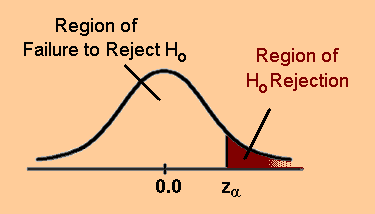Comparing Distributions

## Comparing Distributions: Z Test

One of the whole points in constructing a statistical distribution of some observed phenomena is to compare that distribution with another distribution to see if they are the same or different. Many environmental problems are amenable to this kind of treatment if we wish to detect whether or not humans might be having an impact on some aspect of the environment. If we have data that allows us to determine the distribution of some event before and after human interaction, we can reliably determine if humans have adversely impacted that event. The simplest way to compare two distributions is via the Z-test.

The Z-test

To compare two different distributions one makes use of a tenant of statistical theory which states that

The error in the mean is calculated by dividing the dispersion by the square root of the number of data points.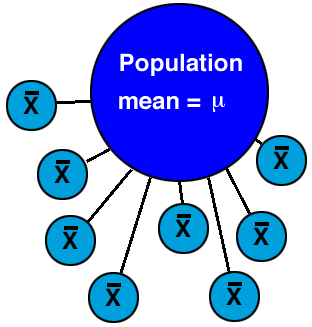In the above diagram, there is some population mean that is the true intrinsic mean value for that population. We can never measure that population directly. Instead, we sample (and we better be randomly sampling) that population by reaching into it (in the above image we have formed 8 separate samples) and getting various samples, each of which are defined by some sample mean:If we have truly randomly sampled the parent population, then there should be no significant differences among the 8 sample means that we have obtained. This is one way you can use to determine, in fact, the likelihood that your sample means it a good indicator of the true population mean.

The error in the mean can be thought of as a measure of how realiable a mean value has been determined. The more samples you have, the more reliable the mean is. But, it goes as the square root of the number of samples! So if you want to improve the reliability of the mean value by, say, a factor of 10, you would have to get 100 times more samples. This can be difficult and often your stuck with what you got. You then have to make use of it.

Ultimately, the purpose of this test is to see if two distributions are significantly different from one another. For instance, the three distributions below do overlap with one another, somewhat – does that mean they are the same or are they different? Remember, statistically significant differences mean that whatever the conditions are that determine one distribution, they are not the same as the conditions that produce the other distribution. (These points will become more clear to you in the second homework assignment when you work through some actual examples.)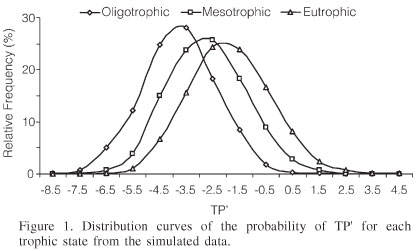The Z-test is especially useful in the case of time series data where you might want to assess a "before and after" comparison of some system to see if there has been effect. One relevant example (which you will do on the second assignment) is to determine whether or not "grade inflation" is real and statistically significant.

It is often said that you can only use the Z-test when you have a sample that you're comparing against a parent population of known mean and standard deviation. This is not really true – you can always use the z-test when comparing two samples.

Comparing Two Sample Means – Find the difference of the two sample means in units of sample mean errors. Difference in terms of significance is: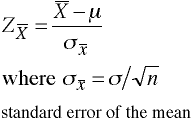But for comparing two samples directly, one needs to compute the Z statistic in the following manner: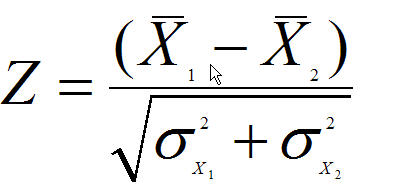Where

• X1 is the mean value of sample one
• X2 is the mean value of sample two
• σx1 is the standard deviation of sample one divided by the square root of the number of data points
• σx2 is the standard deviation of sample two divided by the square root of the number of data points

Here is a specific example of the Z-test application:

Eugene vs. Seattle rainfall comparison over 25 years (so N = number of samples = 25):

Eugene Seattle
mean = 51.5 inches mean = 39.5 inches
dispersion = 8.0 dispersion = 7.0
N = 25 N = 25
error in mean = 8.0/5 error in mean = 7.0/5
error in mean = 1.6 error in mean = 1.4

So far this example:

• X1 = 51.5
• X2 = 39.5
• X1 - X2 = 12
• σx1 = 1.6
• σx2 = 1.4
• sqrt of σx12 + σx22 =sqrt(1.62 + 1.42) = sqrt(2.56 +1.96) = 2.1

Therefore, the Z-statistic is 12/2.1 = 6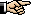which means there is a highly significant difference between these two distributions which means it really does rain significantly more in Eugene than in Seattle.

You can verify these numbers by using the Z-test tool (comparing two means) accessible from the statistical tools area.

In general, in more qualitative terms:

• If the Z-statistic is less than 2, the two samples are the same.

• If the Z-statistic is between 2.0 and 2.5, the two samples are marginally different

• If the Z-statistic is between 2.5 and 3.0, the two samples are significantly different

• If the Z-statistic is more then 3.0, the two samples are highly signficantly different

In many fields of social science, the cricital value of Z (Zcrit) is 2.33 which represents p =0.01 (shaded area below has 1% of the total area of the probability distribution function). This means the two distributions have only a 1% probability of being actual the same.